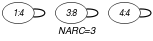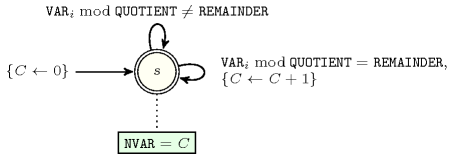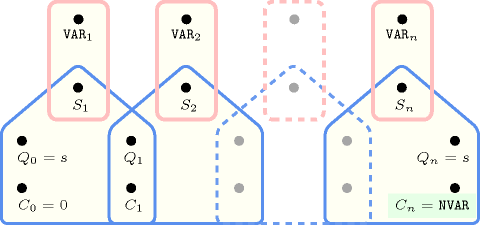## 5.27. among_modulo

Origin

Derived from $\mathrm{𝚊𝚖𝚘𝚗𝚐}$.

Constraint

$\mathrm{𝚊𝚖𝚘𝚗𝚐}_\mathrm{𝚖𝚘𝚍𝚞𝚕𝚘}\left(\mathrm{𝙽𝚅𝙰𝚁},\mathrm{𝚅𝙰𝚁𝙸𝙰𝙱𝙻𝙴𝚂},\mathrm{𝚁𝙴𝙼𝙰𝙸𝙽𝙳𝙴𝚁},\mathrm{𝚀𝚄𝙾𝚃𝙸𝙴𝙽𝚃}\right)$

Arguments
 $\mathrm{𝙽𝚅𝙰𝚁}$ $\mathrm{𝚍𝚟𝚊𝚛}$ $\mathrm{𝚅𝙰𝚁𝙸𝙰𝙱𝙻𝙴𝚂}$ $\mathrm{𝚌𝚘𝚕𝚕𝚎𝚌𝚝𝚒𝚘𝚗}\left(\mathrm{𝚟𝚊𝚛}-\mathrm{𝚍𝚟𝚊𝚛}\right)$ $\mathrm{𝚁𝙴𝙼𝙰𝙸𝙽𝙳𝙴𝚁}$ $\mathrm{𝚒𝚗𝚝}$ $\mathrm{𝚀𝚄𝙾𝚃𝙸𝙴𝙽𝚃}$ $\mathrm{𝚒𝚗𝚝}$
Restrictions
 $\mathrm{𝙽𝚅𝙰𝚁}\ge 0$ $\mathrm{𝙽𝚅𝙰𝚁}\le |\mathrm{𝚅𝙰𝚁𝙸𝙰𝙱𝙻𝙴𝚂}|$ $\mathrm{𝚛𝚎𝚚𝚞𝚒𝚛𝚎𝚍}$$\left(\mathrm{𝚅𝙰𝚁𝙸𝙰𝙱𝙻𝙴𝚂},\mathrm{𝚟𝚊𝚛}\right)$ $\mathrm{𝚁𝙴𝙼𝙰𝙸𝙽𝙳𝙴𝚁}\ge 0$ $\mathrm{𝚁𝙴𝙼𝙰𝙸𝙽𝙳𝙴𝚁}<\mathrm{𝚀𝚄𝙾𝚃𝙸𝙴𝙽𝚃}$ $\mathrm{𝚀𝚄𝙾𝚃𝙸𝙴𝙽𝚃}>0$
Purpose

$\mathrm{𝙽𝚅𝙰𝚁}$ is the number of variables of the collection $\mathrm{𝚅𝙰𝚁𝙸𝙰𝙱𝙻𝙴𝚂}$ taking a value that is congruent to $\mathrm{𝚁𝙴𝙼𝙰𝙸𝙽𝙳𝙴𝚁}$ modulo $\mathrm{𝚀𝚄𝙾𝚃𝙸𝙴𝙽𝚃}$.

Example
$\left(3,〈4,5,8,4,1〉,0,2\right)$

In this example $\mathrm{𝚁𝙴𝙼𝙰𝙸𝙽𝙳𝙴𝚁}=0$ and $\mathrm{𝚀𝚄𝙾𝚃𝙸𝙴𝙽𝚃}=2$ specifies that we count the number of even values taken by the different variables. As a consequence the $\mathrm{𝚊𝚖𝚘𝚗𝚐}_\mathrm{𝚖𝚘𝚍𝚞𝚕𝚘}$ constraint holds since exactly 3 values of the collection $〈4,5,8,4,1〉$ are even.

Typical
 $\mathrm{𝙽𝚅𝙰𝚁}>0$ $\mathrm{𝙽𝚅𝙰𝚁}<|\mathrm{𝚅𝙰𝚁𝙸𝙰𝙱𝙻𝙴𝚂}|$ $|\mathrm{𝚅𝙰𝚁𝙸𝙰𝙱𝙻𝙴𝚂}|>1$ $\mathrm{𝚀𝚄𝙾𝚃𝙸𝙴𝙽𝚃}>1$ $\mathrm{𝚀𝚄𝙾𝚃𝙸𝙴𝙽𝚃}<$$\mathrm{𝚖𝚊𝚡𝚟𝚊𝚕}$$\left(\mathrm{𝚅𝙰𝚁𝙸𝙰𝙱𝙻𝙴𝚂}.\mathrm{𝚟𝚊𝚛}\right)$
Symmetries
• Items of $\mathrm{𝚅𝙰𝚁𝙸𝙰𝙱𝙻𝙴𝚂}$ are permutable.

• An occurrence of a value $u$ of $\mathrm{𝚅𝙰𝚁𝙸𝙰𝙱𝙻𝙴𝚂}.\mathrm{𝚟𝚊𝚛}$ such that $u\mathrm{mod}\mathrm{𝚀𝚄𝙾𝚃𝙸𝙴𝙽𝚃}=\mathrm{𝚁𝙴𝙼𝙰𝙸𝙽𝙳𝙴𝚁}$ (resp. $u\mathrm{mod}\mathrm{𝚀𝚄𝙾𝚃𝙸𝙴𝙽𝚃}\ne \mathrm{𝚁𝙴𝙼𝙰𝙸𝙽𝙳𝙴𝚁}$) can be replaced by any other value $v$ such that $v\mathrm{mod}\mathrm{𝚀𝚄𝙾𝚃𝙸𝙴𝙽𝚃}=\mathrm{𝚁𝙴𝙼𝙰𝙸𝙽𝙳𝙴𝚁}$ (resp. $u\mathrm{mod}\mathrm{𝚀𝚄𝙾𝚃𝙸𝙴𝙽𝚃}\ne \mathrm{𝚁𝙴𝙼𝙰𝙸𝙽𝙳𝙴𝚁}$).

Arg. properties
• Functional dependency: $\mathrm{𝙽𝚅𝙰𝚁}$ determined by $\mathrm{𝚅𝙰𝚁𝙸𝙰𝙱𝙻𝙴𝚂}$, $\mathrm{𝚁𝙴𝙼𝙰𝙸𝙽𝙳𝙴𝚁}$ and $\mathrm{𝚀𝚄𝙾𝚃𝙸𝙴𝙽𝚃}$.

• Contractible wrt. $\mathrm{𝚅𝙰𝚁𝙸𝙰𝙱𝙻𝙴𝚂}$ when $\mathrm{𝙽𝚅𝙰𝚁}=0$.

• Contractible wrt. $\mathrm{𝚅𝙰𝚁𝙸𝙰𝙱𝙻𝙴𝚂}$ when $\mathrm{𝙽𝚅𝙰𝚁}=|\mathrm{𝚅𝙰𝚁𝙸𝙰𝙱𝙻𝙴𝚂}|$.

• Aggregate: $\mathrm{𝙽𝚅𝙰𝚁}\left(+\right)$, $\mathrm{𝚅𝙰𝚁𝙸𝙰𝙱𝙻𝙴𝚂}\left(\mathrm{𝚞𝚗𝚒𝚘𝚗}\right)$, $\mathrm{𝚁𝙴𝙼𝙰𝙸𝙽𝙳𝙴𝚁}\left(\mathrm{𝚒𝚍}\right)$, $\mathrm{𝚀𝚄𝙾𝚃𝙸𝙴𝙽𝚃}\left(\mathrm{𝚒𝚍}\right)$.

Remark

By giving explicitly all values $v$ that satisfy the equality $v\mathrm{mod}\mathrm{𝚀𝚄𝙾𝚃𝙸𝙴𝙽𝚃}=\mathrm{𝚁𝙴𝙼𝙰𝙸𝙽𝙳𝙴𝚁}$, the $\mathrm{𝚊𝚖𝚘𝚗𝚐}_\mathrm{𝚖𝚘𝚍𝚞𝚕𝚘}$ constraint can be modelled with the $\mathrm{𝚊𝚖𝚘𝚗𝚐}$ constraint. However the $\mathrm{𝚊𝚖𝚘𝚗𝚐}_\mathrm{𝚖𝚘𝚍𝚞𝚕𝚘}$ constraint provides a more compact form.

generalisation: $\mathrm{𝚊𝚖𝚘𝚗𝚐}$ (list of $\mathrm{𝚟𝚊𝚕𝚞𝚎𝚜}$ $𝚟$ such that $𝚟\mathrm{mod}\mathrm{𝚀𝚄𝙾𝚃𝙸𝙴𝙽𝚃}$ = $\mathrm{𝚁𝙴𝙼𝙰𝙸𝙽𝙳𝙴𝚁}$ replaced by list of $\mathrm{𝚟𝚊𝚕𝚞𝚎𝚜}$).

Keywords
Arc input(s)

$\mathrm{𝚅𝙰𝚁𝙸𝙰𝙱𝙻𝙴𝚂}$

Arc generator
$\mathrm{𝑆𝐸𝐿𝐹}$$↦\mathrm{𝚌𝚘𝚕𝚕𝚎𝚌𝚝𝚒𝚘𝚗}\left(\mathrm{𝚟𝚊𝚛𝚒𝚊𝚋𝚕𝚎𝚜}\right)$

Arc arity
Arc constraint(s)
$\mathrm{𝚟𝚊𝚛𝚒𝚊𝚋𝚕𝚎𝚜}.\mathrm{𝚟𝚊𝚛}\mathrm{mod}\mathrm{𝚀𝚄𝙾𝚃𝙸𝙴𝙽𝚃}=\mathrm{𝚁𝙴𝙼𝙰𝙸𝙽𝙳𝙴𝚁}$
Graph property(ies)
$\mathrm{𝐍𝐀𝐑𝐂}$$=\mathrm{𝙽𝚅𝙰𝚁}$

Graph model

The arc constraint corresponds to a unary constraint. For this reason we employ the $\mathrm{𝑆𝐸𝐿𝐹}$ arc generator in order to produce a graph with a single loop on each vertex.

Parts (A) and (B) of Figure 5.27.1 respectively show the initial and final graph associated with the Example slot. Since we use the $\mathrm{𝐍𝐀𝐑𝐂}$ graph property, the loops of the final graph are stressed in bold.

##### Figure 5.27.1. Initial and final graph of the $\mathrm{𝚊𝚖𝚘𝚗𝚐}_\mathrm{𝚖𝚘𝚍𝚞𝚕𝚘}$ constraint(a) (b)
Automaton

Figure 5.27.2 depicts the automaton associated with the $\mathrm{𝚊𝚖𝚘𝚗𝚐}_\mathrm{𝚖𝚘𝚍𝚞𝚕𝚘}$ constraint. To each variable ${\mathrm{𝚅𝙰𝚁}}_{i}$ of the collection $\mathrm{𝚅𝙰𝚁𝙸𝙰𝙱𝙻𝙴𝚂}$ corresponds a 0-1 signature variable ${S}_{i}$. The following signature constraint links ${\mathrm{𝚅𝙰𝚁}}_{i}$ and ${S}_{i}$: ${\mathrm{𝚅𝙰𝚁}}_{i}\mathrm{mod}\mathrm{𝚀𝚄𝙾𝚃𝙸𝙴𝙽𝚃}=\mathrm{𝚁𝙴𝙼𝙰𝙸𝙽𝙳𝙴𝚁}⇔{S}_{i}$.

##### Figure 5.27.2. Automaton of the $\mathrm{𝚊𝚖𝚘𝚗𝚐}_\mathrm{𝚖𝚘𝚍𝚞𝚕𝚘}$ constraint##### Figure 5.27.3. Hypergraph of the reformulation corresponding to the automaton (with one counter) of the $\mathrm{𝚊𝚖𝚘𝚗𝚐}_\mathrm{𝚖𝚘𝚍𝚞𝚕𝚘}$ constraint: since all states variables ${Q}_{0},{Q}_{1},\cdots ,{Q}_{n}$ are fixed to the unique state $s$ of the automaton, the transitions constraints share only the counter variable $C$ and the constraint network is Berge-acyclic